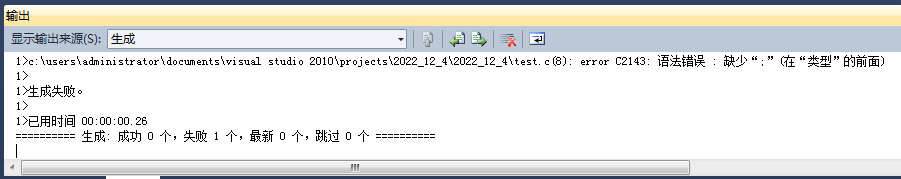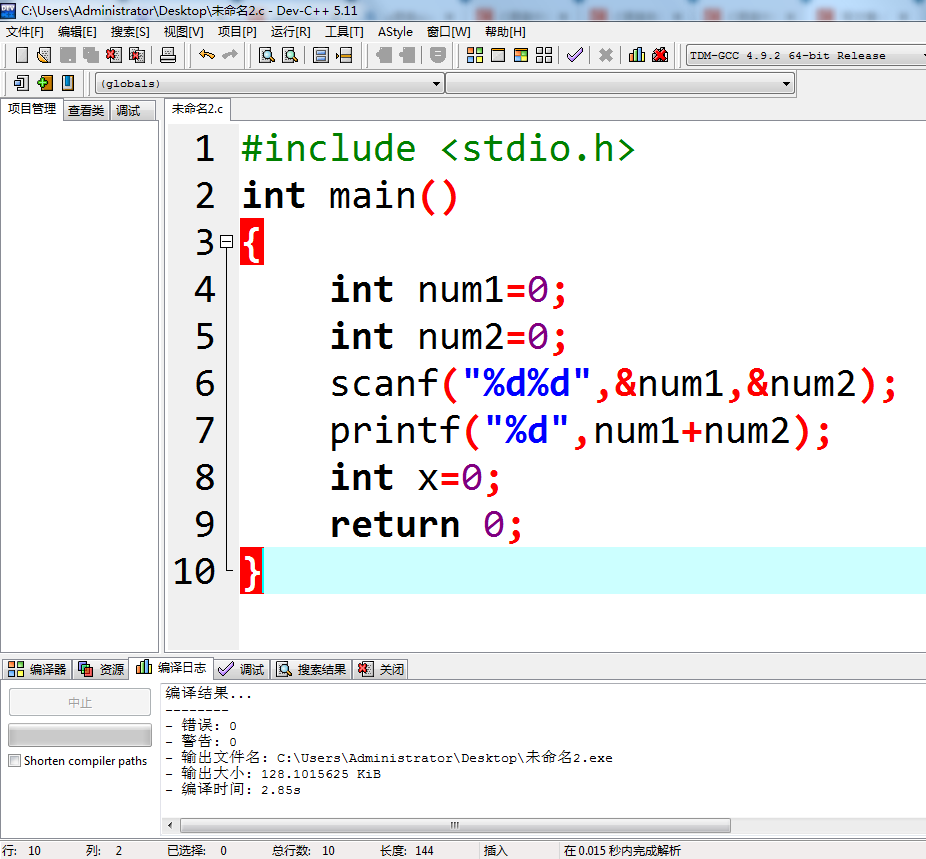# 凡是过往，皆为序章

C语言编程题大家都做对了多少呢？

Stay Hungry, Stay Foolish.

C语言初阶系列先从《初识C语言》讲起，在《初识C语言》中只是基本了解C语言的基础知识，对C语言有一个大概的认识。每个知识点都只是简单认识不做仔细讲解，在后边的章节中会细讲。

?局部变量和全局变量

# 什么是C语言

C语言广泛地用于底层开发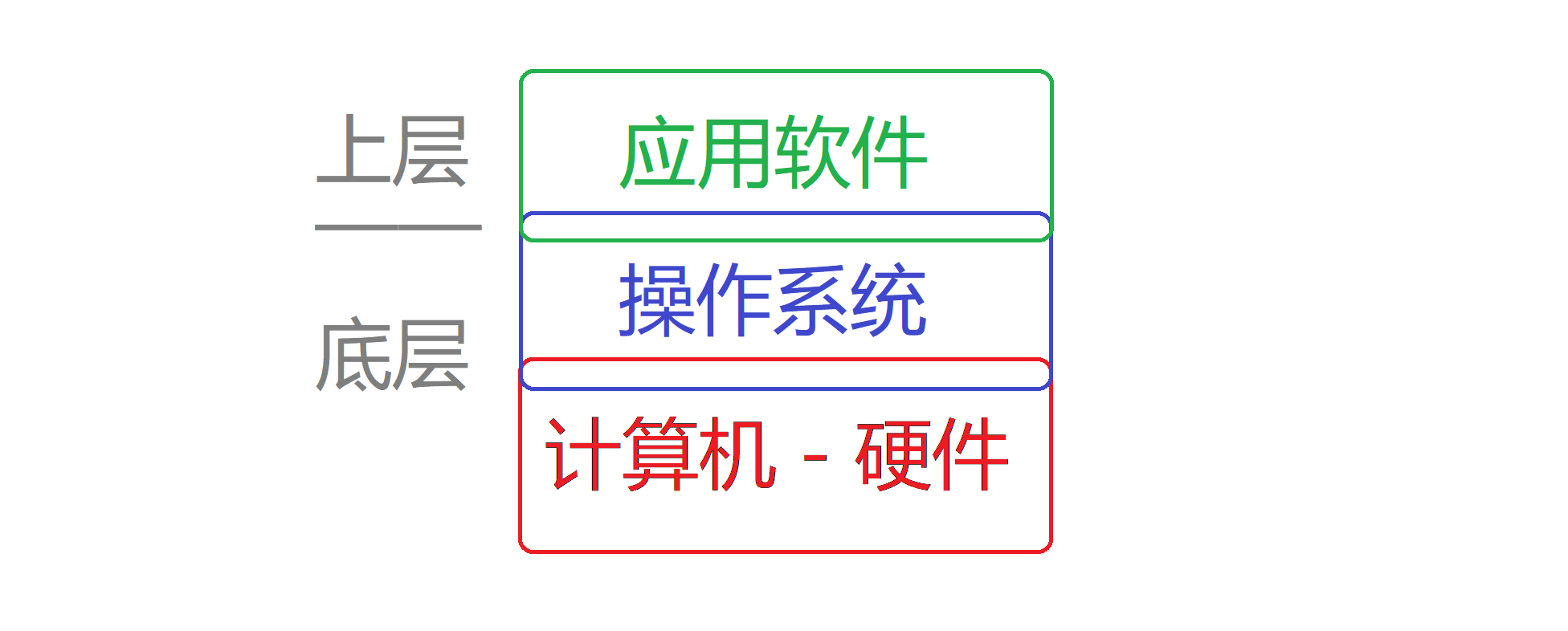000101 - SUB

C语言标准应运而生。C语言的编译器主要有Clang、GCC、MSVC、Turbo C、DevC++等。

Clang是苹果公司的，GCC在Linux上用得比较多，MSVC是最常用的微软的编译器。

VS2013及更高得版本都可以。

Visual Studio里集成了编辑器、编译器、链接器、调试器，既能编辑代码，又能编译代码，又能链接代码，又能调试代码。它把各种功能集成在一起，所以叫集成开发环境。

# 第一个C语言程序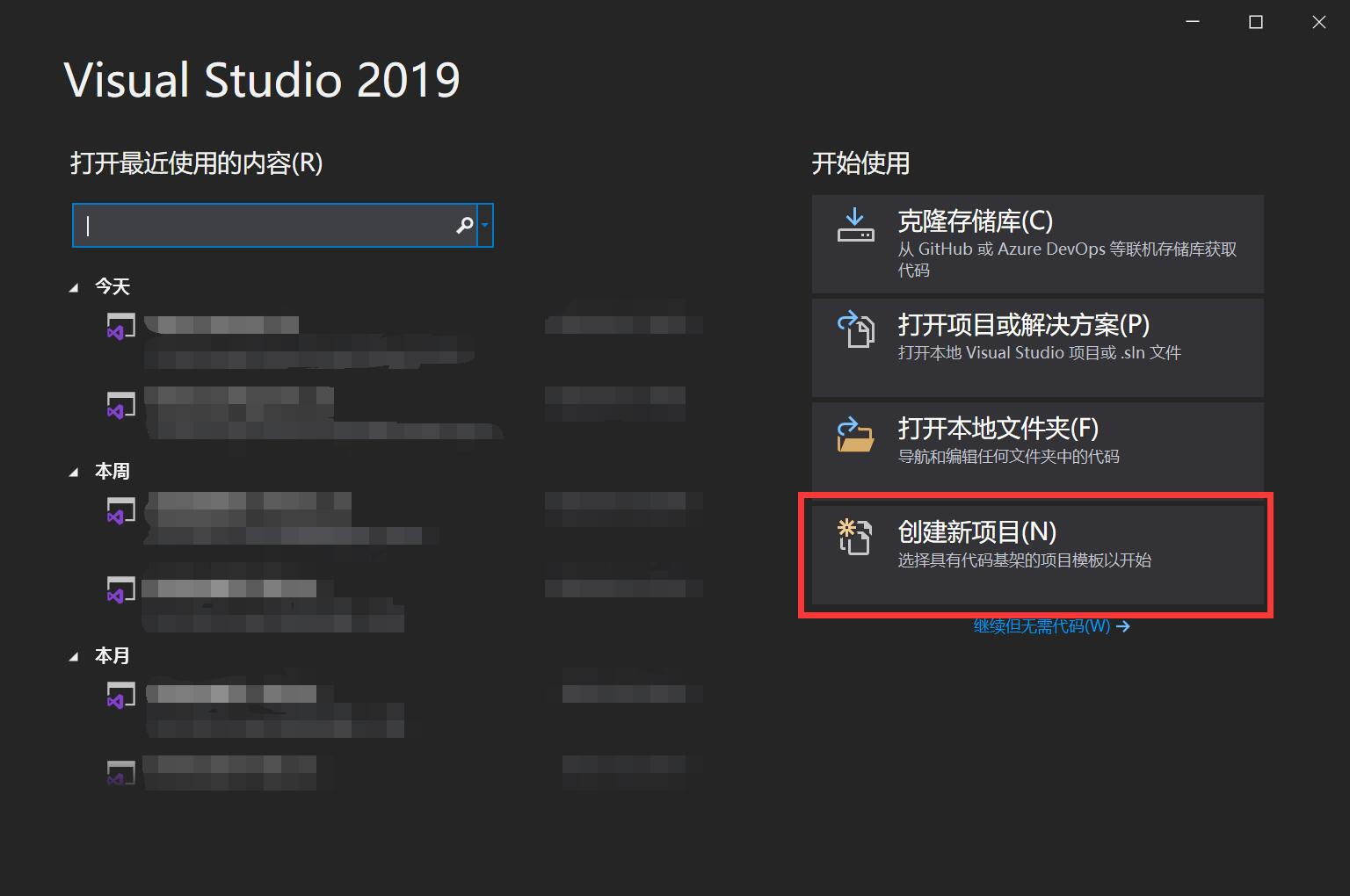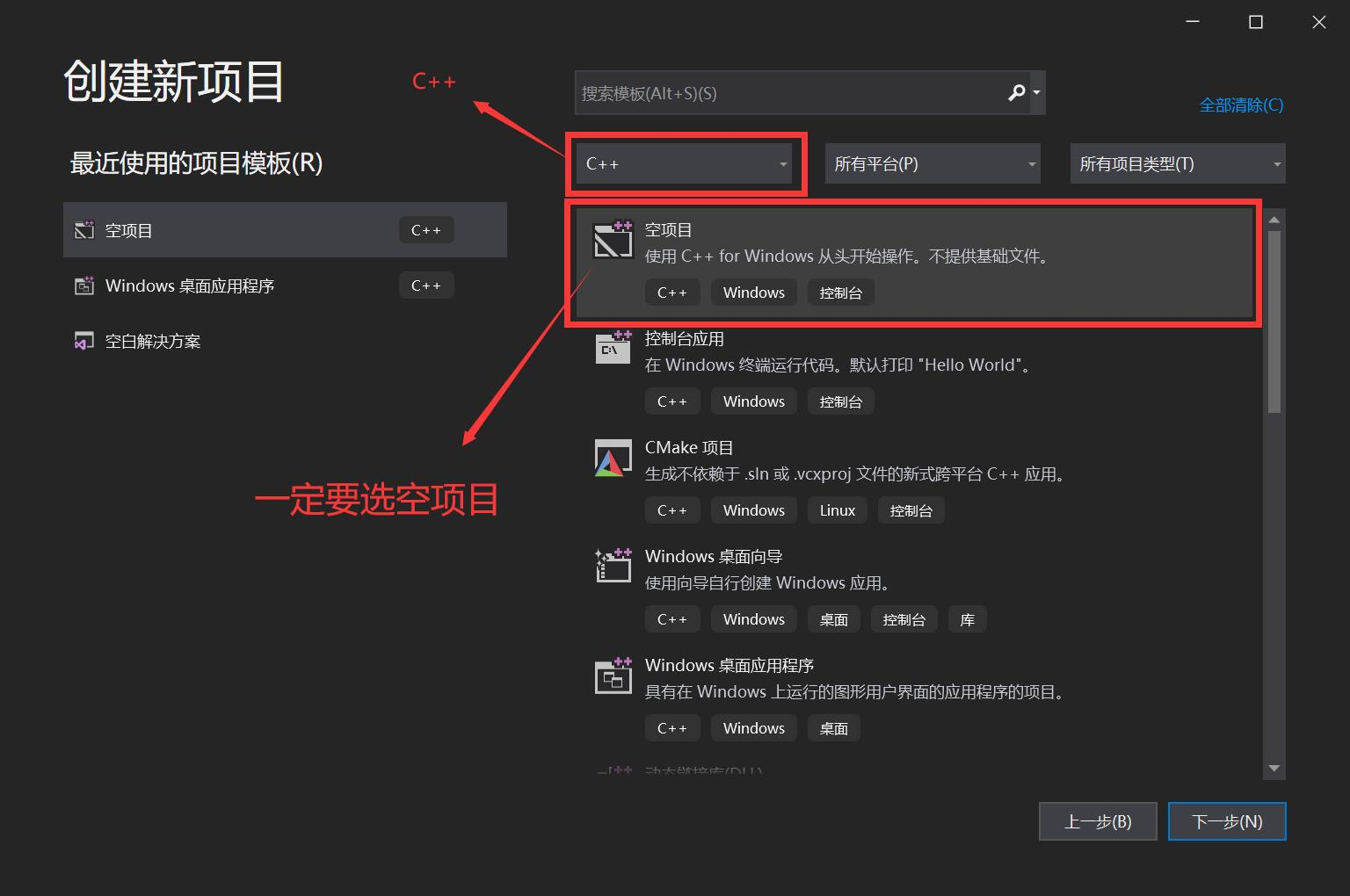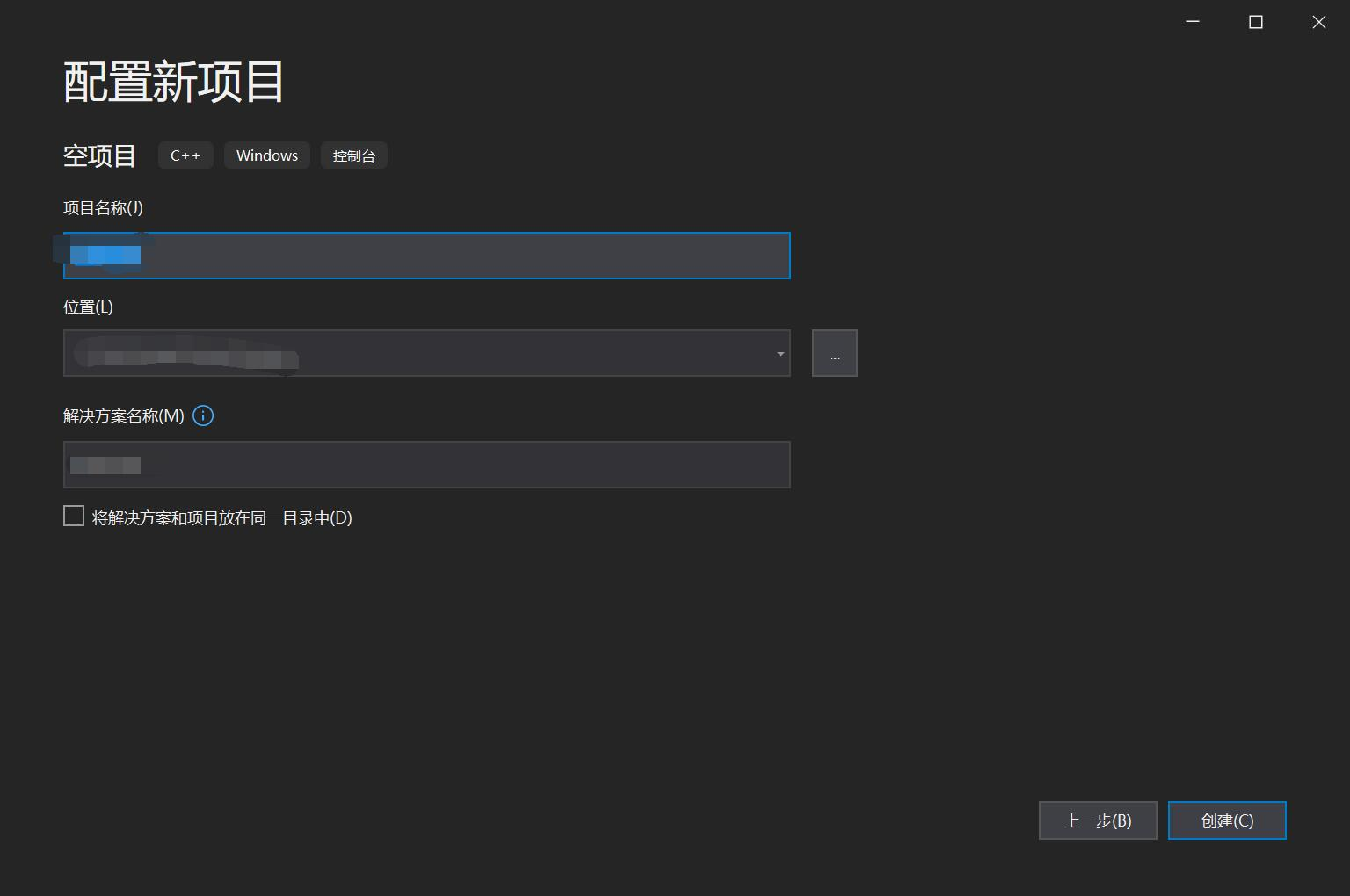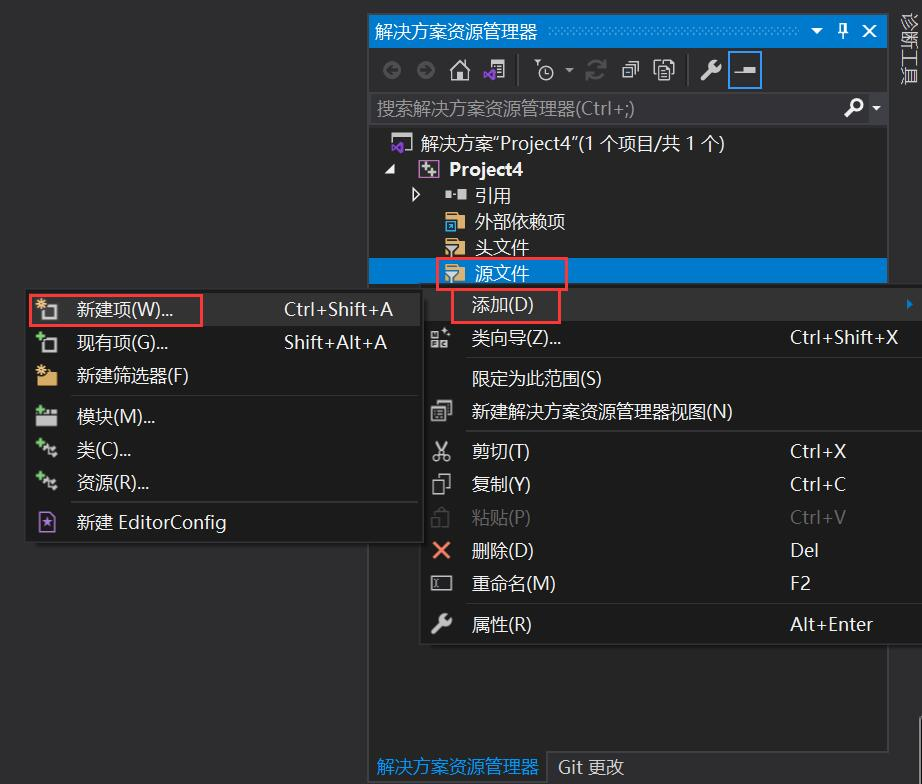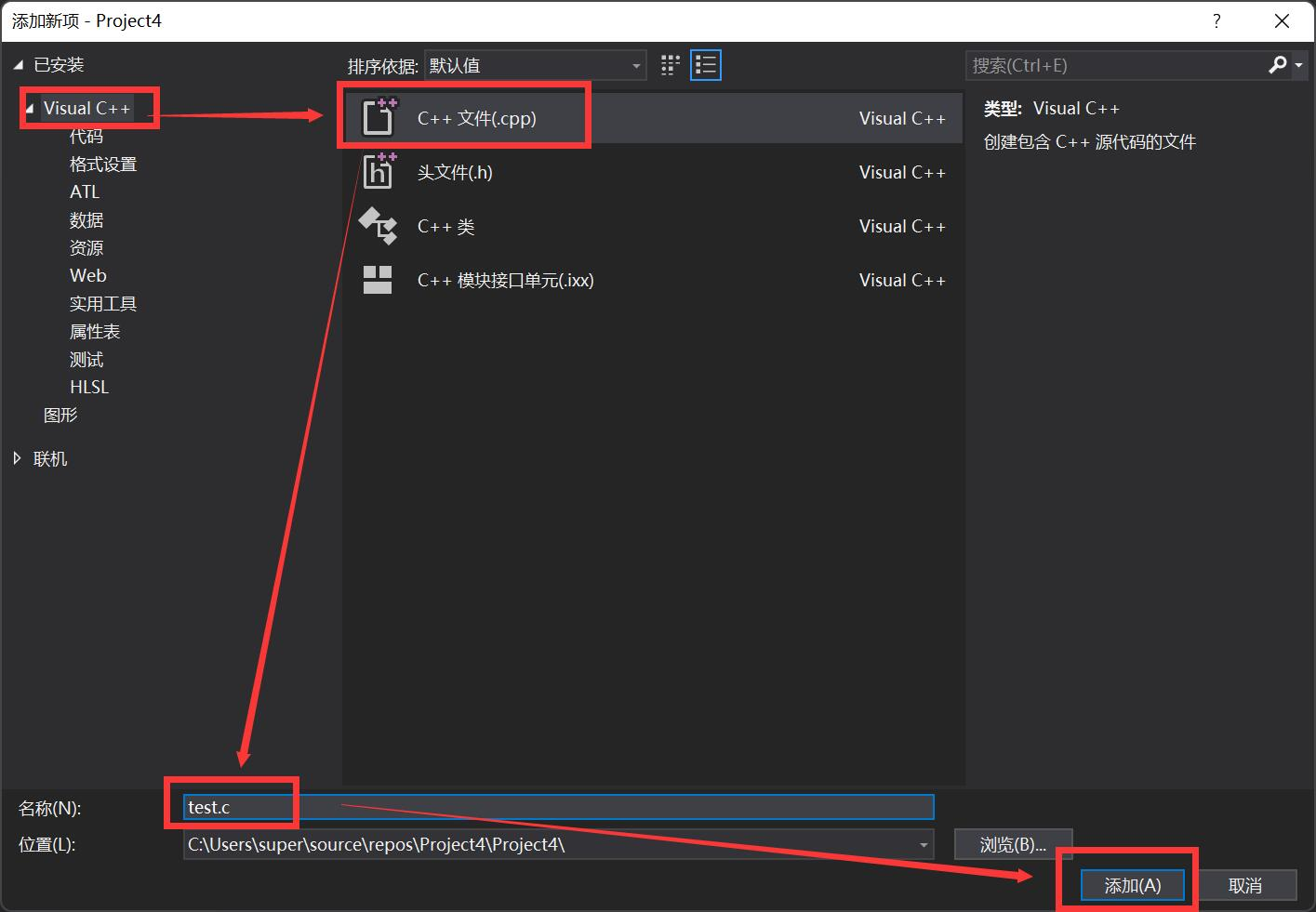?点击添加就可以完成C语言文件的创建了。

``#define _CRT_SECURE_NO_WARNINGS 1``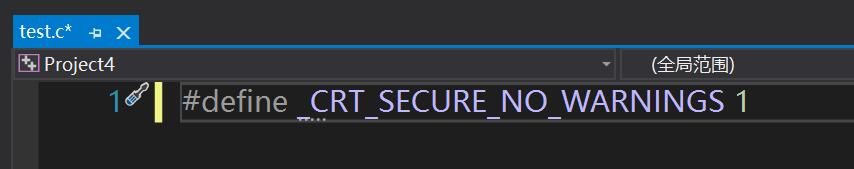``````int main()
{

return 0;
}``````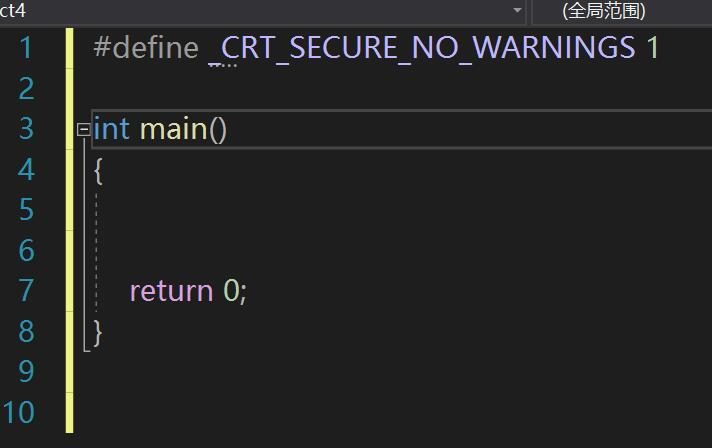``````void main()
{

}``````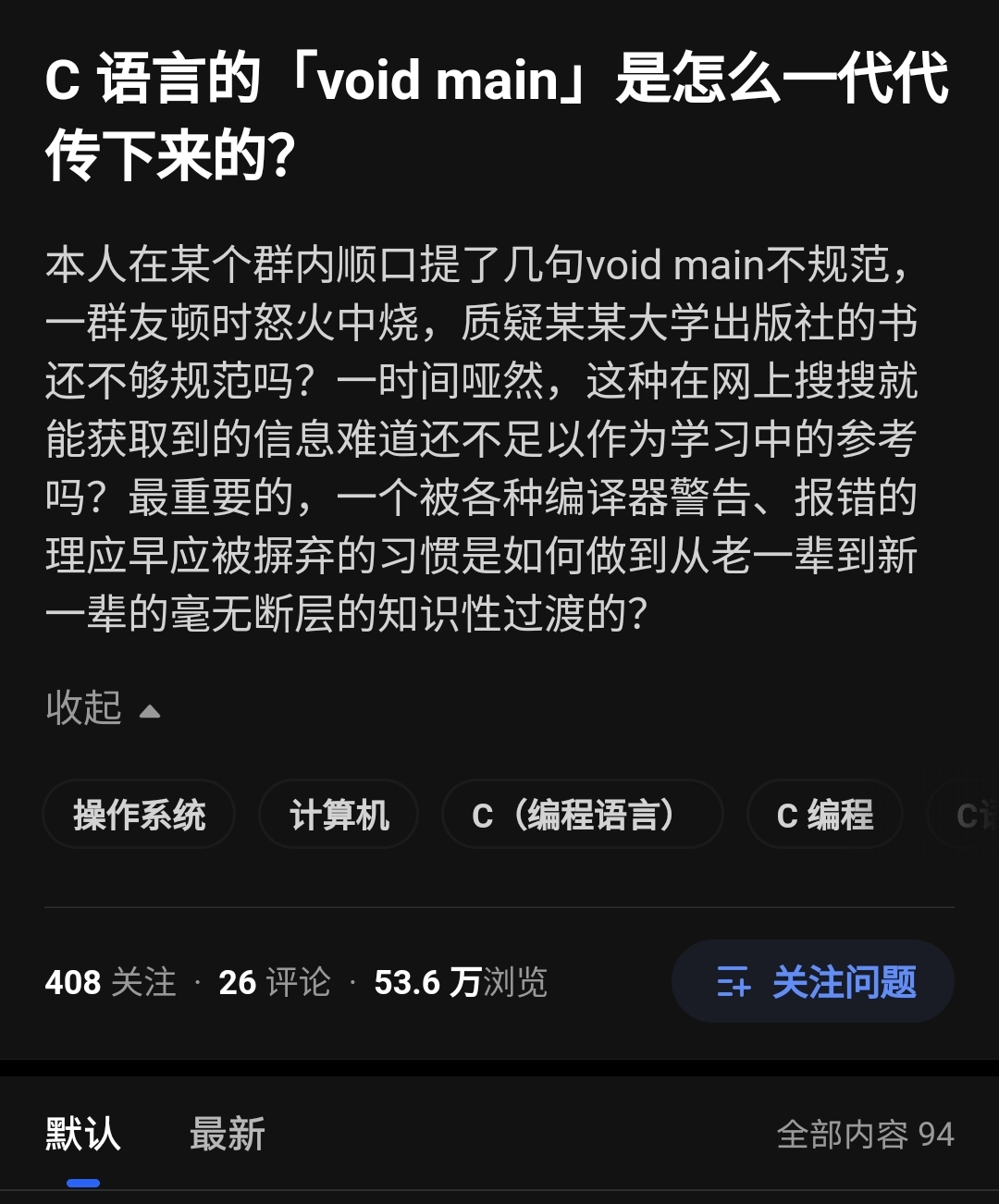``````int main(void)
{

return 0;
}``````

main后边的括号里写void，说明这个主函数不需要参数。

``````int main(int argc,char* argv[])
{

return 0;
}``````

return 0代表这个函数返回的值是0，整数0。

int是整型的意思，说明这个函数返回的值是整型的。

``````#include <stdio.h>
int main()
{
printf("Hello World!");
return 0;
}``````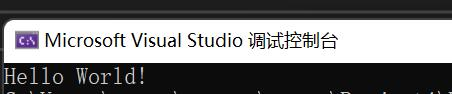include是包含的意思，在C语言中意思为下面的代码包含stdio.h头文件。

stdio是什么？是缩写，全称是standard input output，”标准输入输出“。

``````#include <stdio.h>
int main()
{
int num1=0;
int num2=0;
scanf("%d%d",&num1,&num2);
printf("%d",num1+num2);
return 0;
}``````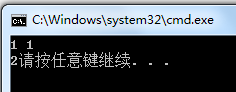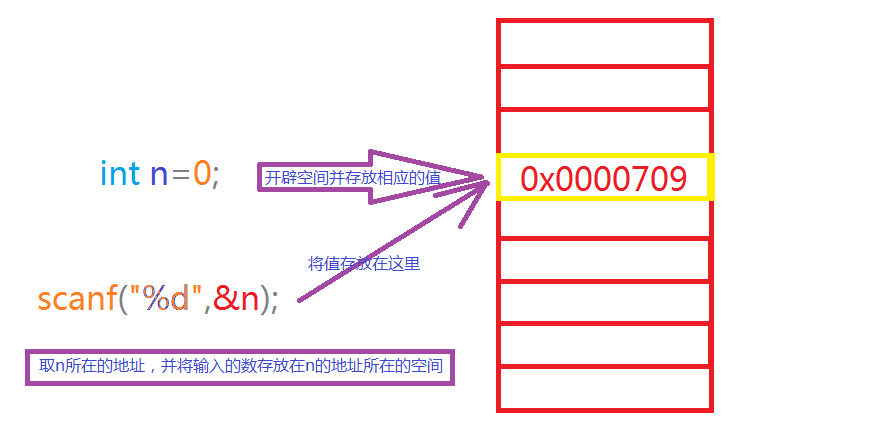``````#include <stdio.h>
int main()
{
printf("Hello World!");
printf("Hello World!");
printf("Hello World!");
printf("Hello World!");
printf("Hello World!");
printf("Hello World!");
return 0;
}``````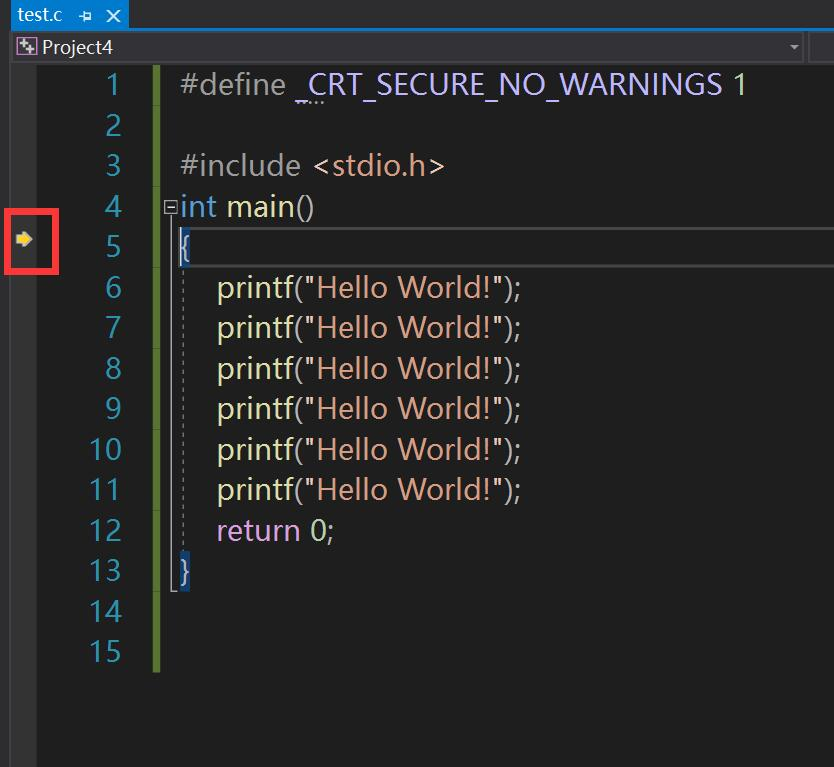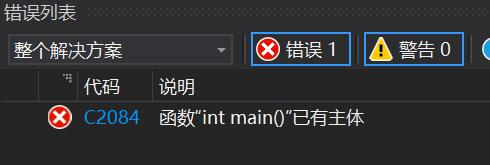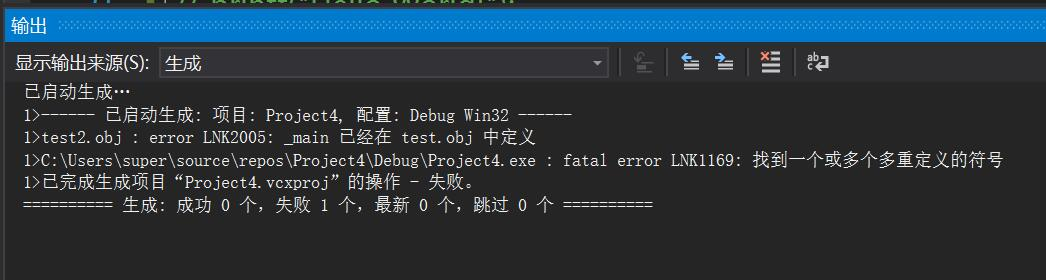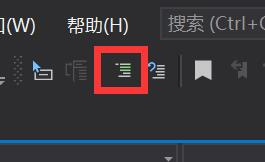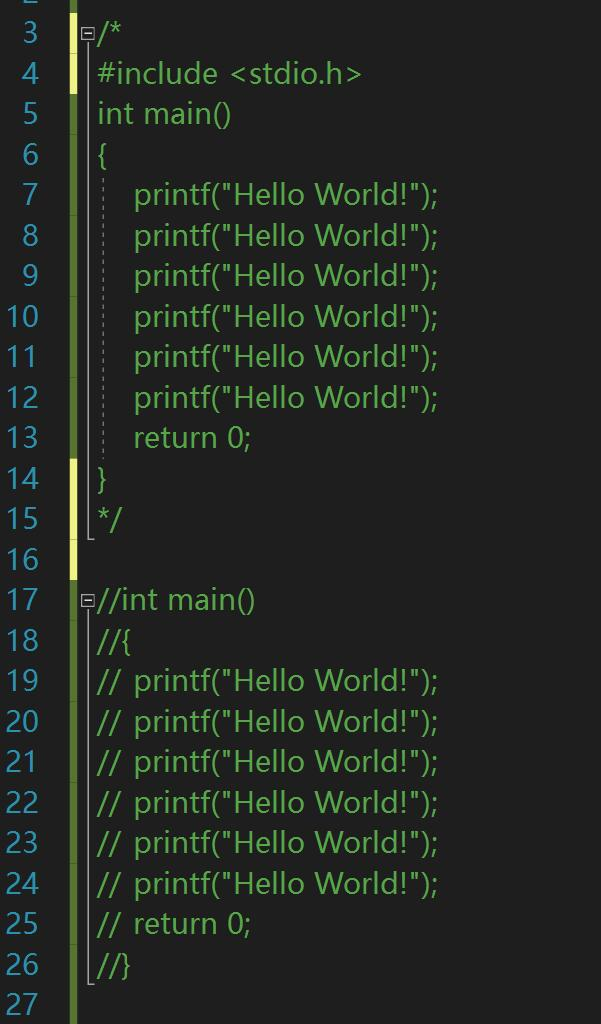# 数据类型

C语言的数据类型有这些：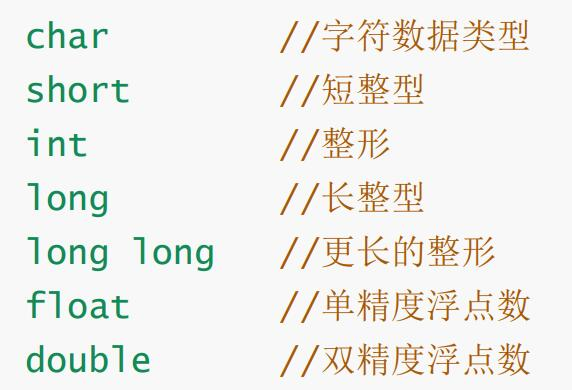C生万物，那块吸铁石是C语言编写的！?

``````#include <stdio.h>
int main()
{
printf("%d",100);
return 0;
}``````

``````#include <stdio.h>
int main()
{
int a = 100;
int b = 200;
printf("%d%d",a,b);
printf("%d%d",a,b);
return 0;
}``````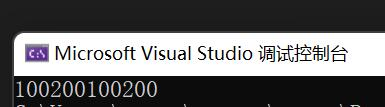``````#include <stdio.h>
int main()
{
int a = 100;
int b = 200;
printf("%d %d",a,b);
return 0;
}``````

?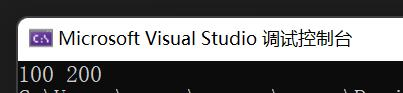``````#include <stdio.h>
int main()
{
int a = 100;
int b = 200;
printf("%d%d\n", a, b);
printf("%d%d\n", a, b);
return 0;
}``````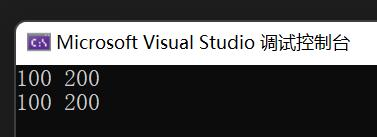char是字符型，一个字符型变量存放一个字符。

``````#include <stdio.h>
int main()
{
char a='A';
printf("%c",a);
return 0;
}``````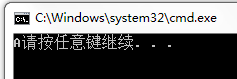``````#include <stdio.h>
int main()
{
char a='AB';
printf("%c",a);
return 0;
}``````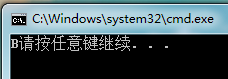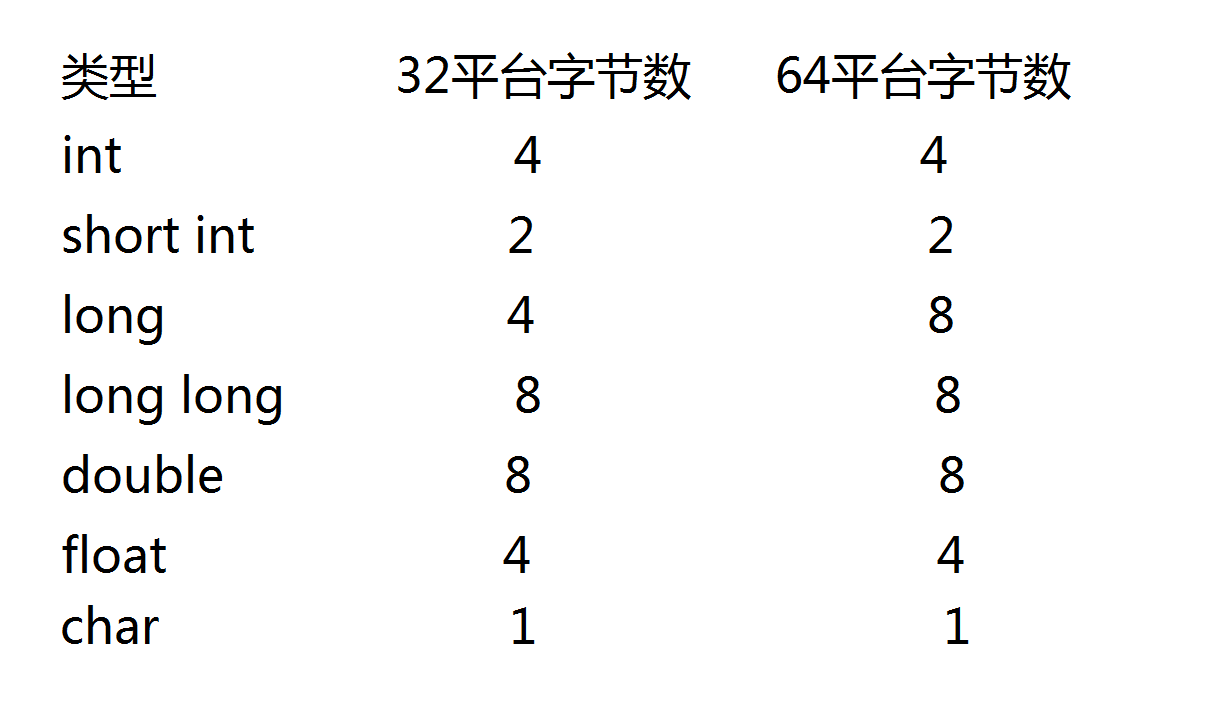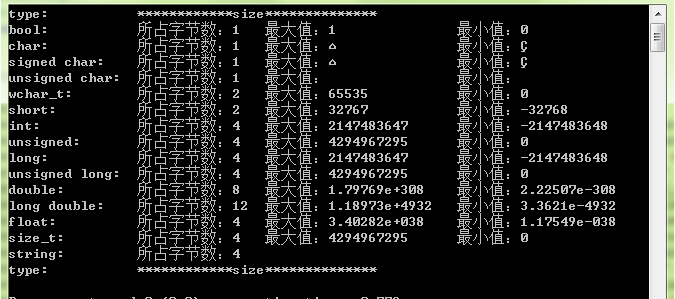``````#include <stdio.h>
int main()
{
char a='A';
int b=0;
long c=0;
printf("%d\n",sizeof(a));
printf("%d\n",sizeof(char));
printf("%d\n",sizeof(b));
printf("%d\n",sizeof(int));
printf("%d\n",sizeof(c));
printf("%d\n",sizeof(long));
return 0;
}``````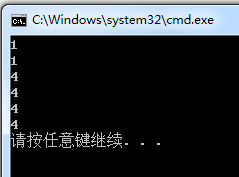``````#include <stdio.h>
int main()
{
int num=5;
char a='A';
double master=93.9;
float king=8844.43;
printf("%d\n",num);
printf("%c\n",a);
printf("%lf\n",master);
printf("%f\n",king);
return 0;
}``````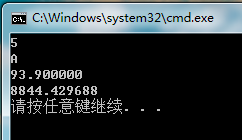?可以看到，正是因为用了不同的字母，打印后才能得到我们所期望的值。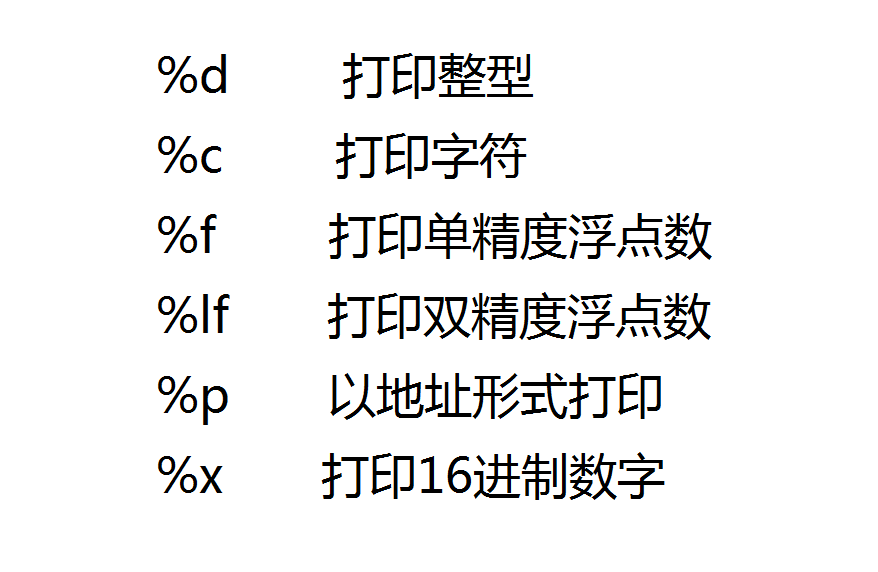# 变量、常量

``````	int age=18;
float weight=45.5f;
char ch='w';``````

1.不能以数字开头

2.只能由字母、数组和下划线组成

3.不能与C语言中的关键字重合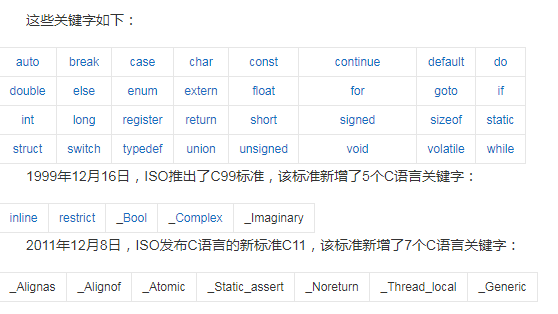## ?局部变量和全局变量

``````#include <stdio.h>
int num=666;
int main()
{
int num=888;
printf("%d",num);
return 0;
}``````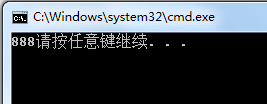?所以说局部变量和全局变量的名称最好不要相同，容易引起误会，产生bug。

``````#include <stdio.h>
int main()
{
{
int num=888;
}
printf("%d",num);
return 0;
}``````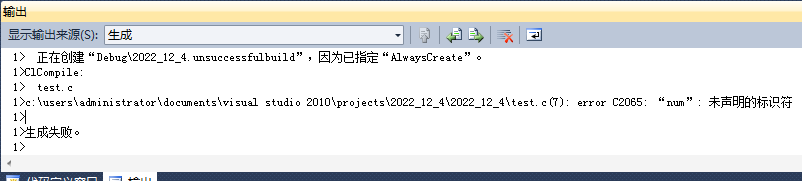C语言语法规定，变量要定义在当前代码块的最前端。

``````int main()
{
int num1=0;
int num2=0;
scanf("%d%d",&num1,&num2);
printf("%d",num1+num2);
int x=0;
return 0;
}``````